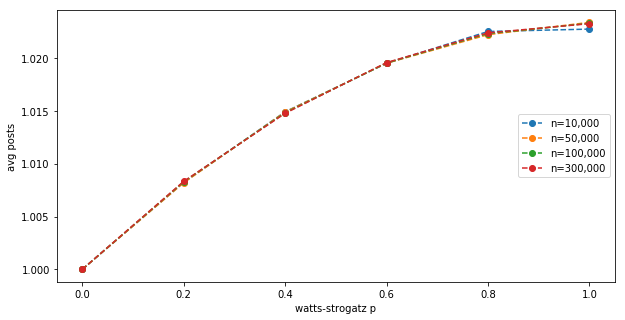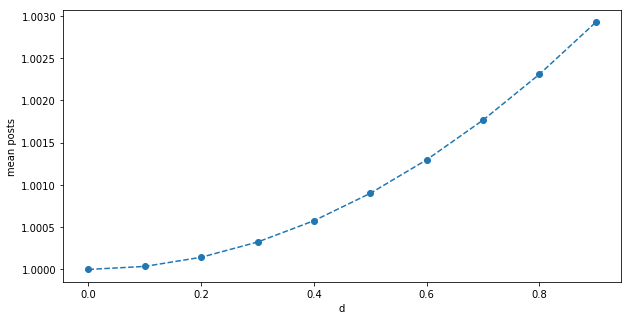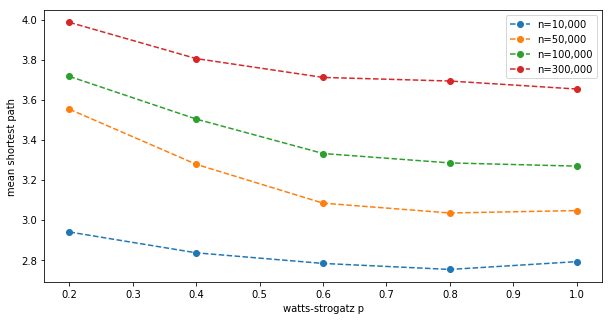# A/B testing and networks effects

Marton Trencseni - Sat 21 March 2020 - Data

## Introduction

In the previous post I calculated the expected average posts on a social network. The model had 2 components:

• intrinsic: users intrinsically create posts
• network effects: users create more posts if they see their friends’ posts

But, there is actually a third, even more nuanced effect: the strength of the network effect depends on the degree distribution in the network.

The code shown below is up on Github.

## Degree dependence of the network effect

Simulations showed that even a weak network effect boosts overall post production significantly. If $c_{int}$ is intrinsic post production, and for each friend's post users create an additional $c_{net}$ post the next day, and on average users have $k$ friends, then in the steady state $c = c_{int} + c \times c_{net} \times k$. Solving this we get $c = \frac{ c_{int} }{ 1 - c_{net} \times k }$.

In the last post, I used a $U(0, 1)$ random variable to multiply both $c_{int}$ and $c_{net}$. This is not neccessary and just introduces noise, so let’s leave it out. For this post let’s use $c_{int}=1/4$ and $c_{net}=3/200$, with these values $c=1$.

In the above formula, the structure of the graph only appears in the parameter $k$. This is a good first approximation, but it’s not entirely accurate. A more accurate way to think about it is to write $c = c_{int} + c \times f(c_{net}, g)$, where $f$ is some function, and $g$ is the graph ($f=c_{net} \times k$ is our initial approximation). Let's explore this dependence further.

We are using Watts-Strogatz graphs for these experiment, which have a re-wiring randomization parameter $p$. When the Watts-Strogatz graph is constructed, initially a ring graph is created (large diameter) with $k$ edges from each node, and then each edge is randomly re-wired with probability $p$ to get a small-world network (small diameter). If we set $p=0$, the degree distribution of the graph is exactly $k$. As we increase $p$, the degree distribution becomes a gaussian around $k$. Code to visualize this:

Note: some of these simulations take a while to run, so I now use multiprocessing. This makes the code harder to read, but the speed-up is significant.

def mp_degree_distribution(args):
(n, k, p) = args
g = connected_watts_strogatz_graph(n, k, p)
ds = Counter(sorted([d for n, d in g.degree()], reverse=True))
d, c = zip(*ds.items())
return p, d, c

n=100*1000
k=50

results = mp_map(
network_scaling_worker.mp_degree_distribution,
[(n, k, p/100.0) for p in range(10, 110, 20)])

plt.figure(figsize=(10,5))
plt.xlabel('degree')
plt.ylabel('node count')
for x in results:
plt.plot(x, x)
plt.legend(['p=%s' % x for x in results])
plt.show()


Shows:Now comes the interesting bit. Let’s calculate the average post production for a Watts-Strogatz graph for different parameters $(n, p)$, with fixed $k=50$:

def mp_lifts(args):
(n, k, p, N, T) = args
g = connected_watts_strogatz_graph(n, k, p).to_directed()
population_A = set(sample(g.nodes, N))
stats = compute_stats(g, population_A, T) # see the previous post
return (args, stats)

ns = [10*1000, 50*1000, 100*1000, 300*1000]
k=50
ps = range(0, 120, 20)
T=50
N=0

results = []
for n in ns:
print('Running simulations for n=%s...' % n)
start_time = time.time()
results.extend(mp_map(
network_scaling_worker.mp_lifts,
[(n, k, p/100.0, N, T) for p in ps],
))
elapsed_time = time.time() - start_time
print('Done! Elapsed %s' % time.strftime("%M:%S", time.gmtime(elapsed_time)))


Shows:This shows the (third) effect:

• At $p=0$, when all nodes have exactly $k$ neighbours, the mean post production is exactly 1.
• At higher $p$, there is an additional boost to post production, due to some nodes having more neighbours than $k$; and this boost is not canceled by some nodes having less neighbours.
• The maximum boost due to uneven degree distribution is about 2.5% at $p=1$ for $k=50$.
• The effect does not seem to depend on the $n$ network size.

This has implications for A/B testing: when we compute the lift in our simulations, we need to use this adjusted baseline.

Another way to get a sense for this is: assume half the nodes are have degree $k=50-d$, half have $k=50+d$ after randomization, so the mean is still $k=50$. We can take the original formula $c = \frac{ c_{int} }{ 1 - c_{net} \times k }$ and make an improved version: $c(d) = \frac{1}{2} ( \frac{ c_{int} }{ 1 - c_{net} \times (k+d) } + \frac{ c_{int} }{ 1 - c_{net} \times (k-d) } )$. This is not the exact formula (the experimental result above is concave, and this is convex), but it’s good for intuition:Initially I suspected the effect is due to the graph diameter decreasing with increasing $p$, so I checked how the mean shortest path depends on $p$ and $n$:

def mp_shortest_paths(args):
(n, k, p, num_samples) = args
g = connected_watts_strogatz_graph(n, k, p)
samples = []
for _ in range(num_samples):
st = sample(g.nodes, 2)
l = nx.shortest_path_length(g, st, st)
samples.append(l)
mean_shortest_path = np.mean(samples)
return (args, mean_shortest_path)

ns = [10*1000, 50*1000, 100*1000, 300*1000]
k=50
ps = range(0, 120, 20)
num_samples = 1000

results = []
for n in ns:
print('Running simulations for n=%s...' % n)
start_time = time.time()
results.extend(mp_map(
network_scaling_worker.mp_shortest_paths,
[(n, k, p/100.0, num_samples) for p in ps],
))
elapsed_time = time.time() - start_time
print('Done! Elapsed %s' % time.strftime("%M:%S", time.gmtime(elapsed_time)))


Shows:This does not appear to be the reason for the additional boost. The mean shortest path in the graph strongly depends on $n$, as expected, but the effect does not. Intuitively, since all nodes are equal for now (there is no A population producing more content), path length shouldn’t matter.

So, to get more accurate lift readings for our A/B tests, we need to first calculate the correct baseline mean posts for a Watts-Strogatz graph with those $(k, p)$ params (no strong $n$ dependence, experimentally, as we just saw):

n=100*1000
k=50
p=0.1
T=50

g = connected_watts_strogatz_graph(n=n, k=k, p=p).to_directed()
posts = prev_posts = None
for t in range(T):
posts = step_posts(g, prev_posts)
prev_posts = posts
baseline_avg_posts = np.mean(list(posts.values()))
print("Correct baseline for a (%s, %s, %s) Watts-Strogatz graph after %s steps = %.4f" % (n, k, p, T, baseline_avg_posts))


Prints:

Correct baseline for a (100000, 50, 0.1) Watts-Strogatz graph after 50 steps = 1.0044


## A/B testing

Let’s re-run the A/B test experiment from the last post and calculate the lifts, but compared to the new, corrected baseline. Let’s have an experimental group of $N$ people, whose intrinsic post production $c_{int}$ is lifted by 5%, using both $N=100$ and $N=1,000$:

ns = [5*1000, 10*1000, 50*1000, 100*1000]
k=50
p=0.1
T=50
num_simulations = 50

params = chain(
prepend(100,  ns),
prepend(1000, ns),
)

def thread_count(n):
if n <= 100*1000: return 24
if n == 200*1000: return 12
else:             return 8

stats_list = []
if __name__ ==  '__main__':
for N, n in params:
print('Running simulations for n=%s...' % n)
start_time = time.time()
results = mp_map(
network_scaling_worker.mp_lifts,
[(n, k, p, N, T) for _ in range(num_simulations)],
thread_count(n),
)
avg_stats = [np.mean([x[i] for x in results]) for i in range(len(results))]
stats_list.append((N, n, avg_stats))
elapsed_time = time.time() - start_time
print('Done! Elapsed %s' % time.strftime("%M:%S", time.gmtime(elapsed_time)))What we expect to see:

• as $N/n$ goes to 0, the rest and overall lifts tend to 0.
• as $N/n$ goes to 1, we expted to see a bigger lift for population A (if $N=n$, we released to 100% and lifted everybody's post production)

## Conclusion

A simple toy post production model on a Watts-Strogatz graph shows multiple interesting effects (also see previous post):

• network effect: boosts post production by a significant factor
• degree distribution effect: the network effect boost is a function of the graph's degree distribituion, which for a Watts-Strogatz graph is a function of $p$
• dampening effect: we underestimate the true intrinsic lift of the A/B test, because A’s non-A friends don’t get the intrinsic post production boost, so As don’t get the boost “back” through these edges
• spillover effect: we measure a lift due to the network effect for friends of A, and further down the network, depending on the distance from As
• clustering effect: if the A group is more tightly clustered, we measured a higher lift
• experiment size effect: as $N/n$ goes to 1, the effect size approaches the true effect size